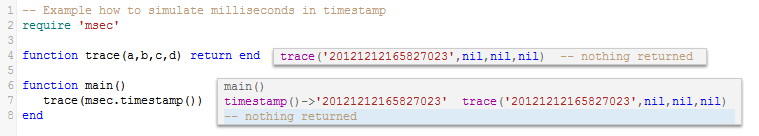# How to build a restartable counter to memorize given state.

• Iguana Translator users, who require precise to millisecond timestamps, had notice that Lua time module offered with Iguana doesn’t allow for milliseconds to be reported. This has its own reasons beyond focus of this discussion.

This example code in this article demonstrates how to use a persistent counter to simulate a count of milliseconds.

The Lua time function os.time() only returns a time value to the nearest second. There are many occasions where milliseconds would be useful to distinguish between timed events. The code in this article solves this problem by simulating milliseconds, and concatenating them to the current time.

Warning! If real timed milliseconds required then please see suggestion at the bottom of this page. The “millisecond values” in example on this page are not timed milliseconds, they are just a sequential count from 1-999 within a second. But this example demonstrates how one could build a restartable counter to memorize given state.

How it works

The code uses a counter as a surrogate for milliseconds. The counter value is appended to the time returned by the os.time()command, and returned as a string. Should processing yield more than 999 newly generated timestamps within less than one second, the code will sleep until current second rolls over to next second and the milliseconds counter resets itself.

The magic is all done in the msec module, all that is needed to do is to call the msec.timestamp() function, as shown:The “milliseconds”, in this case “023”, have been appended to the time.

Sample Code

Code for main():

```-- Example how to simulate milliseconds in timestamp
require 'msec'

function trace(a,b,c,d) return end

function main()
trace(msec.timestamp())
end```

Code for the msec module:

```msec ={}

SQLITE_DB ='test.sqlite'
conn = db.connect{
api=db.SQLITE,
name=SQLITE_DB
}

local function zfill(s,N)
if s:len() < 3 then
repeat
s = '0' .. s
until s:len() == 3
end
return s
end

local function Msec()

-- the only configurable parameter is name of table 't'
local t = 'milliseconds'

local function createTable()
local Sql='drop table if exists '..t
conn:execute{sql=Sql,live=true}
Sql = "CREATE TABLE IF NOT EXISTS "..t.." \
('msec' INT(255) NULL, 'sec' INT(255) NULL);"
conn:execute{sql=Sql,live=true}
end

local function tableExists()

local rs=conn:query('SELECT name FROM sqlite_master')

local function foo(i)
if rs[i].name:nodeValue()
== t  then
return true
end
end

for i=1,#rs do
if foo(i) then return true end
end
end

local function currentSec()
local rs=conn:query('SELECT * FROM '..t)
if rs.sec:nodeValue()
== os.date("%S",os.time()) then
return true
end
end

local function currentMsec()
local rs=conn:query('SELECT * FROM '..t)
return rs.msec:nodeValue()
end

local function writeMsec(msec)
conn:execute{
sql="INSERT INTO "..t.." \
(msec,sec) VALUES ("..msec..",\
"..os.date("%S",os.time())..");",
live=true
}
end

local function updateMsec(msec)
conn:execute{
sql="UPDATE "..t.." \
set msec="..msec..",\
sec="..os.date("%S",os.time())..";",
live=true
}
end

if tableExists() then
local ms=currentMsec()
local msnext=ms+1
if ms=='999' then
repeat
util.sleep(1)
until os.date("%S",os.time())~=currentSec()
updateMsec(1)
return 1
else
updateMsec(msnext)
return msnext
end
else
createTable()
writeMsec(1)
return 1
end

end

function msec.timestamp()
local v = Msec()
v=zfill(tostring(v),3)
local t = os.date("%Y%m%d%H%M%S",os.time())
return t..v
end```

What's Next?

The above example shows how to use a counter to simulate milliseconds. Just remember these are not timed milliseconds, just a count from 1-999 within a second.

If timed milliseconds needed, then solution is different. Please use OS independent solution, a call to os.clock(), as explained in this example.

You must be logged in to reply to this topic.